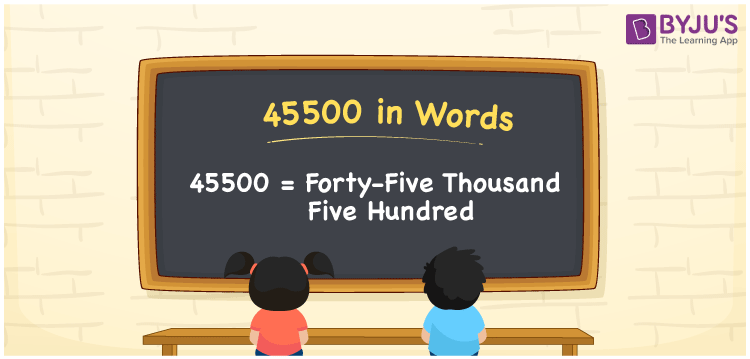# 45500 in Words

45500 in words can be expressed as Forty-Five Thousand Five Hundred. We know that the number name of any number can be written with the help of a place value chart. It is important to determine the place of each digit while converting the numbers to words. 45500 is a cardinal number as it depicts an exact quantity. Let us learn how to spell and write the number 45500 in English using a place value chart.

 45500 in words Forty-Five Thousand Five Hundred Forty-Five Thousand Five Hundred in numerical form 45500

## 45500 in English Words

Generally, we write numbers in words in Mathematics using the English alphabet. Thus, 45500 in words is read as Forty-Five Thousand Five Hundred.## How to Write 45500 in Words?

45500 can be written in words using the place value of a number. First, find the place value of each digit of a given number before writing the number name for 45500. Here, 45500 has five digits. Let us design a place value chart up to five digits.

 Ten-Thousands Thousands Hundreds Tens Ones 4 5 5 0 0

Thus, we can write the expanded form as:

4 x Ten Thousand + 5 x Thousand + 5 x Hundred + 0 × Ten + 0 × One

= 4 x 10000 + 5 x 1000 + 5 x 100 + 0 + 0

= 40000 + 5000 + 500

= 45500

= Forty-Five Thousand Five Hundred

Hence, 45500 in words is written as Forty-Five Thousand Five Hundred

Interesting way of writing 45500 in words

4 = Four

45 = Forty-Five

455 = Four Hundred and Fifty-Five

4550 = Four Thousand Five Hundred Fifty

45500 = Forty-Five Thousand Five Hundred

Hence, the word form of the number 45500 is Forty-Five Thousand Five Hundred

45500 is a natural number that is succeeded by 45499 and preceded by 45501

• 45500 in words – Forty-Five Thousand Five Hundred
• Is 45500 an odd number? – No
• Is 45500 an even number? – Yes
• Is 45500 a perfect square number? – No
• Is 45500 a perfect cube number? – No
• Is 45500 a prime number? – No
• Is 45500 a composite number? – Yes

## Frequently Asked Questions on 45500 in Words

Q1

### How do you write 45500 in words?

45500 in words is written as Forty-Five Thousand Five Hundred.
Q2

### Express the value of 60000 – 14500 in words.

Simplifying 60000 – 14500, we get 45500. Therefore, 45500 in words is Forty-Five Thousand Five Hundred.
Q3

### Is 45500 a composite number?

Yes, 45500 is a composite number.